Milne method

A finite-difference method for the solution of the Cauchy problem for systems of first-order ordinary differential equations: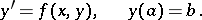The method uses the finite-difference formulawhere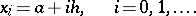In computations using this formula it is necessary, by some other means, to find an additional initial value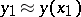. The Milne method has second-order accuracy and is Dahlquist stable, that is, all solutions of the homogeneous difference equation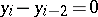are bounded uniformly with respect tofor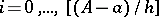, for any fixed interval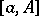. For Dahlquist stability it is sufficient that the simple roots of the characteristic polynomial of the left-hand side of the difference equation do not exceed one in modulus, and that the multiple roots are strictly less than one in modulus. In the case given, the characteristic polynomial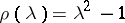has roots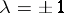and, consequently, the stability condition holds. However, in solving a system of equations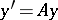with a matrixhaving negative eigen values, a rapid growth in the calculation error occurs.

The predictor-corrector Milne method uses a pair of finite-difference formulas:

a predictor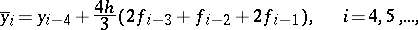and a corrector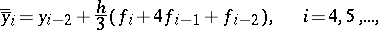whereAn approximate expression for the error is given by the quantityAdditional initial values,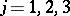, are calculated by some other means, for example, by the Runge–Kutta method, which has fourth-order accuracy. The method was proposed in .